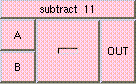SUBTRACTTHE BOX'S MAIN HELP: This is the subtraction function. The result is A - B. A and B can be scalars, vectors, or colors. The result will be a scalar if both A and B are scalars. The result will be a vector if either A and B are vectors. The result will be a color if either A and B are colors. A type mis-match will result from the subtraction of vectors and colors. A (input): This is the value to be decreased. The input may be a scalar, vector, or color. However a type mis-match will result from the subtraction of vectors and colors. B (input): This is the decrement. The input may be a scalar, vector, or color. However a type mis-match will result from the subtraction of vectors and colors. OUT (output): This is the subtraction function. The result is A - B. A and B can be scalars, vectors, or colors. The result will be a scalar if both A and B are scalars. The result will be a vector if either A and B are vectors. The result will be a color if either A and B are colors. A type mis-match will result from the subtraction of vectors and colors.

<-- BACK TO Math